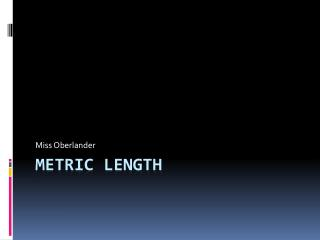# Metric Length - PowerPoint PPT PresentationDownload PresentationMetric Length

Metric Length
Download Presentation## Metric Length

- - - - - - - - - - - - - - - - - - - - - - - - - - - E N D - - - - - - - - - - - - - - - - - - - - - - - - - - -
##### Presentation Transcript

1. Miss Oberlander Metric Length

2. Metric Length is based on a meter. • Meter Stick (a little longer than a yard)

3. Millimeter  1,000mm = 1 m mm to measure about a spot on a ladybug

4. Centimeter  100cm = 1 m cm is about the length of a thumbtack

5. Meter (slightly longer than yd) m can measure the height of a small easel

6. Kilometer  1,000 meters

7. Other countries measure distance with kilometers instead of miles! • Check out the speed limit signs from around the world! It looks like you can drive faster…but remember a km is a little bit more than ONLY ½ mi.

8. Kilometers measure metric distance.

9. Game! • Hold up the sign language letter for the correct answer for each slide!

10. The length of a ladybug? Ounces Grams Millimeters

11. The length of a car? Tons Meters Kilograms

12. The length of a frog? • Grams • Meters • Centimeters

13. The length of a cat’s tail? • Pounds • Millimeters • Centimeters

14. The length of dress up wings? • Grams • Millimeters • Meters

15. The length of a cadet hat? • Grams • Centimeters • millimeters

16. The mass of a bumblebee? • Grams • Millimeters • Ounces

17. The length of a Macbook Pro? • Pounds • Millimeters • Centimeters

18. The weight of a small kitten? • Ounces • Grams • Millimeters

19. The weight of a tiny teacup? • Ounces • Grams • Millimeters

20. The length from here to the nearest train station? • Kilometers • Centimeters • Kilograms

21. The weight of light hat? • Grams • Ounces • Millimeters

22. The length of a hat? • Centimeters • Meters • Kilometers

23. The length of a pier? • Centimeters • Meters • kilometers

24. The height of a lighthouse? • Kilometers • Meters • Centimeters

25. The length of a dress? • Yards • Centimeters • Pounds

26. The length of a bookcase? • Feet • Centimeters • Kilograms

27. The length of a bicycle trip? • Feet • Kilometers • Tons

28. The length of a hike/walk along the coast? • Tons • Kilograms • Kilometers

29. The length of a piece of cheesecake? • Grams • Centimeters • Meters

30. Exit Ticket Time: Directions: For the next 5 questions, write the letter of your choice on the index card next to the number. Write your name on it and put it into the green bin.

31. Which of these measures length? • Grams • Pounds • Centimeters

32. Which of these measures height? • Kilograms • Tons • Meters

33. Which of these measures weight? • Millimeters • Ounces • Grams

34. Which of these measures mass? • Kilometers • Kilograms • Pounds

35. #5. Is a mile or a kilometer longer?